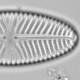## english::as.english

It is conventional to write small integers as words rather than figures in text, especially if they are at the start of the sentence. This caused me some grief with rmarkdown, which I have started using for presentations, papers and blog-posts: my code gave me figures, I wanted words. Then I found the `english` package.

```library("english")
as.english(42)
```
```##  forty two
```
```as.english(-1)
```
```##  minus one
```

We need to be careful if we use this inline with

```The Ultimate Answer to Life, The Universe and
Everything is...`r as.english(42)`
```

as this will be converted back to a figure.

The Ultimate Answer to Life, The Universe and Everything is…42.

Wrapping the command in `as.character` will fix this

````r as.character(as.english(2))` to the power of
`r as.character(as.english(276709))` to
`r as.character(as.english(1))` against
```

two to the power of two hundred and seventy six thousand seven hundred and nine to one against

It would be a cunning plan to make a function to run `as.character(as.english(x))` if it is needed repeatedly.

Of course, sometimes we will want to start a sentence with a number and need the first letter capitalising. This can be done with regular expressions (otherwise known as magic).

````r gsub("(^)(.)", "\\1\\U\\2", as.english(42), perl = TRUE)`
```

Forty two

As was hinted above by the conversion back to figures, objects made by `as.english` retain their numeric values, and so can be used in calculations

```char.english <- function(x)as.character(as.english(x))
num <- lapply(0:9, as.english)
names(num) <- sapply(0:9, char.english)# set names
attach(num) #make available

six * nine
```

```##  fifty four
```Ecologist with interests in quantitative methods and palaeoenvironments
This entry was posted in R and tagged . Bookmark the permalink.

### 1 Response to english::as.english

1.richard telford says:

This wrapper function will convert integers to a word and capitalise as requested

```as.eng <- function(x, capitalise = FALSE){
e <- as.character(english::as.english(x))
if(capitalise){
gsub("(^)(.)", "\\1\\U\\2", e, perl = TRUE)
}
e
}
```